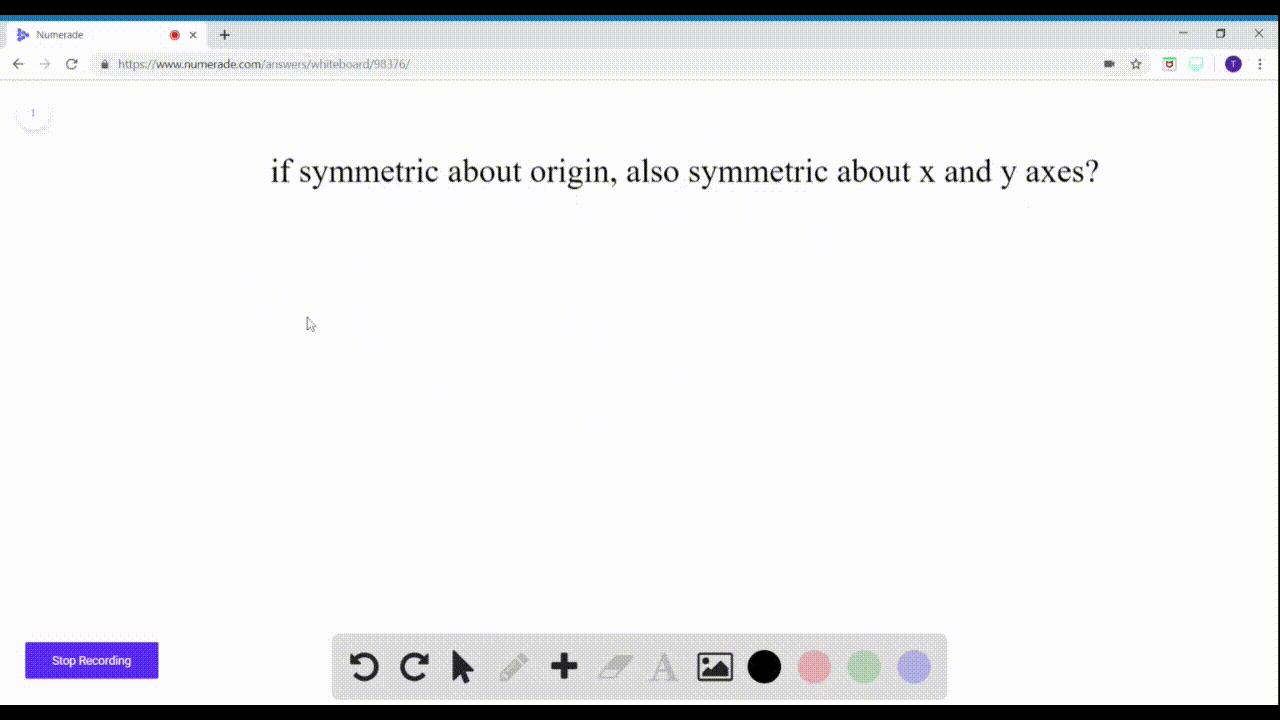🎉 The Study-to-Win Winning Ticket number has been announced! Go to your Tickets dashboard to see if you won! 🎉View Winning Ticket### $7-8=$ Yes or No? If $N o,$ give a reason. If th…

02:24Problem 7

# $7-8=$ Yes or $N o ?$ If $N o,$ give a reason.If the graph of an equation is symmetric with respect to boththe $x$ - and $y$ -axes, is it necessarily symmetric with respect tothe origin?

## Discussion

You must be signed in to discuss.

## Video Transcript

this question asks if graph of of an equation symmetric with respect both the X and Y axes Is it necessarily symmetric with respect to the origin? And the answer is yes. Let me explain if the graph oven equation is symmetric with respect to the X axis and that means that for a given point a B point a negative B is also on the graph. If it is symmetric with respect to the Y axis, then for that given point a b the point negative A B is also on the axis. No note, please, for symmetry with respect to the origin. If the point a b is on the graph than the point negative, a negative B is on the graph noticed that both conditions from the X and Y axis symmetry tests are involved in the origin symmetry, so therefore the answer is yes.